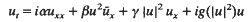Hostname: page-component-797576ffbb-lm8cj Total loading time: 0 Render date: 2023-12-06T05:47:30.475Z Has data issue: false Feature Flags: { "corePageComponentGetUserInfoFromSharedSession": true, "coreDisableEcommerce": false, "useRatesEcommerce": true } hasContentIssue false

# On smooth solutions to the initial value problem for the mixed nonlinear Schrödinger equations*

Published online by Cambridge University Press:  14 November 2011

## Synopsis

Solutions to the initial value problem for the mixed nonlinear Schrödinger equationare considered. Conditions on the constants α,β, γ, function g(·) and initial data u(x, 0) are given so that, for this problem, the unique existence of smooth solutions is proved. In addition, the decay behaviours of the smooth solutions as |x|→+∞ are discussed.

Type
Research Article

## Access options

Get access to the full version of this content by using one of the access options below. (Log in options will check for institutional or personal access. Content may require purchase if you do not have access.)

## References

1Rogister, A.. Phys. Fluids 14 (1971), 27332739.Google Scholar
2Chen, H. H., Lee, Y. C. and Lin, C. S.. Phys. Scr. 20 (1979), 490495.Google Scholar
3Ablowitz, M. J., Ramani, A., Segur, H.. A connection between nonlinear evolution equations and ordinary differential equations of P-type. II, J. Math. Phys. 21 (1980), 10061015.Google Scholar
4Gerdjikov, V. S. and Ivanov, I.. Bulg. J. Phys. 10 (1983), 130134.Google Scholar
5Kundu, A.. Landau–Lifshitz ad higher-order nonlinear systems gauge generated from nonlinear Schrödinger type equations, J. Math. Phys. 25 (1984), 34333436.Google Scholar
6Chen, Y. M.. The initial-boundary value problem for a class of nonlinear Schrödinger equations, Acta Math. Sci. 6 (1986), 405ö418.Google Scholar
7Tsutsumi, M. and Fukuda, I.. On solutions of the derivative nonlinear Schrödinger equation, I. Funkcial. Ekvac. 23 (1980), 259277.Google Scholar
8Tsutsumi, M. and Fukuda, I.. On solutions of the derivative nonlinear Schrödinger equation, II. Funkcial. Ekvac. 24 (1981), 8594.Google Scholar
9Guo, B. L.. Galerkin Finite Element Method and Error Estimates for the system of Multi-dimensional Higher-order Generalized BBM-KDV Equations, Kexue Tongbao 28 (1983), 310315.Google Scholar
10Zhou, Y. L.. Nonlinear P.D.E. in Applied Science, U.S. Japan Seminar, Tokyo, 1982.Google Scholar
11Lin, C. S.. Interpolation inequalities with weights, Comm. Partial Differential Equations 11 (1986), 15151538.Google Scholar
12Klainerman, S.. Global esistence for nonlinear wave equations. Comm. Pure Appl. Math. 33 (1980), 43101.Google Scholar
13Guo, B. L.. Proceedings of the 1980 Beijing symposium on Differential Geometry and Differential Equation 3 (1982), 12271246.Google Scholar
14Kaup, D. J. and Newell, A. C.. An exact solution for a derivative nonlinear Schrödinger equation, J. Math. Phys. 19 (1978), 798801.Google Scholar